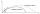# Planimetrics - math word problems

Study plane measurements, including angles, distances, and areas. In other words - measurement and calculation of shapes in the plane. Perimeter and area of plane shapes.

#### Number of problems found: 1947

• AP RT triangleThe length of the sides of a right triangle form an arithmetic progression, longer leg is 24 cm long. What are the perimeter and area?
• Triangular pyramidA regular tetrahedron is a triangular pyramid whose base and walls are identical equilateral triangles. Calculate the height of this body if the edge length is a = 8 cm
• Surface area 6Find the surface area of a prism whose bases are right triangles with sides of length 3, 4, and 5 inches and a height of 8 inches. Include a sketch
• Parallelogram - sideCalculate the length of the side of a parallelogram whose area is 175 dm2 and height to that side is 24.2 dm long.
• Cuboid and eq2Calculate the volume of cuboid with square base and height 6 cm if the surface area is 48 cm2.
• Diamond area from diagonalsIn the diamond ABCD is AB = 4 dm and the length of the diagonal is 6.4 dm long. What is the area of the diamond?
• Parallelogram ABCDThe area of parallelogram ABCD is 440 cm2. Points M and N are the midpoints of the sides AB and BC. What is the area of a quadrilateral MBND?
• Hypotenuse and heightIn a right triangle is length of the hypotenuse c = 56 cm and height hc = 4 cm. Determine the length of both trangle legs.
• Cable car 2Cable car rises at an angle 41° and connects the upper and lower station with an altitude difference of 1175 m. How long is the track of cable car?
• Ballistic curveThe ballistic grenade was fired at a 45° angle. The first half ascended, the second fall. How far and how far it reached if his average speed was 1200km/h, and 12s took from the shot to impact.
• Square prismCalculate the volume of a square prism of high 2 dm wherein the base is: rectangle with sides 17 cm and 1.3 dm
• The sizeThe size of a Trapezium are 3/4×cm, ×cm 2(×+1)cm and 3(×+2)cm long respectively if it's perimeter is 60cm, calculate the length of each side.
• Arc-sectorarc length = 17 cm area of sector = 55 cm2 arc angle = ? the radius of the sector = ?
• Isosceles trapezoidCalculate the area of an isosceles trapezoid whose bases are in the ratio of 4:3; leg b = 13 cm and height = 12 cm.
• Construct rhombusConstruct rhombus ABCD if given diagonal length | AC | = 8cm, inscribed circle radius r = 1.5cm
• Rotating cone IICalculate area of surface of rotating cone with base radius r=19 cm and height h=9 cm.
• Tripled squareIf you tripled the length of the sides of the square ABCD you increases its content by 200 cm2. How long is the side of the square ABCD?
• ChordIn a circle with radius r=60 cm is chord 4× longer than its distance from the center. What is the length of the chord?
• Two diagonalsThe diagonals of the diamond EFGH have lengths in the ratio 1: 2. What is the circumference of a rhombus if the longer of the diagonals is 8 cm long?
• Triangle KLMIn the rectangular triangle KLM, where is hypotenuse m (sketch it!) find the length of the leg k and the height of triangle h if hypotenuse's segments are known mk = 5cm and ml = 15cm

Do you have an interesting mathematical word problem that you can't solve it? Submit a math problem, and we can try to solve it.

We will send a solution to your e-mail address. Solved examples are also published here. Please enter the e-mail correctly and check whether you don't have a full mailbox.

Please do not submit problems from current active competitions such as Mathematical Olympiad, correspondence seminars etc...